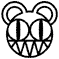# EXAMPLES OF INVARIANTS:

## THE KNOT GROUP

One useful invariant is called the knot group.  Given a knot K, if we fix a point b somewhere in the complement S of K (i.e., somewhere in the three-dimensional space outside the knot), and draw paths from b to b, each traveling through S, we can form a set of paths P.  Note that two elements p1 and p2 of P are equivalent if p1 can be deformed into p2.  That is, p1 may be pulled, pushed, crossed over itself, but not broken or moved out of S.  We can form a class called [p1] containing all the elements of P which are equivalent to p1.  The set P of all different classes [p1], where p1 is in P, can be shown to be an algebraic group, which we will call the knot group.  Understanding this requires a background in Abstract Algebra, and I will show this here.

Showing that the knot group is an invariant is not hard.  I formulate a moderately rigorous proof using ideas from Neurwith: Take a knot K, with complement S and knot group P.  Then assume that K can be transformed into an equivalent knot K` with complement S` and knot group P`.  Take some class of elements [p] of P, and show that [p] is also in P`.  During deformation of K into K`, [p] is transferred from S to S`, because S is only deformed, not broken, to form S`.   Hence, [p] is in P`.  The same argument holds for transformation from K` to K.  Therefore, given a knot K, the element [p] of the knot group of K will always remain the same after deformation of K into any knots which are equivalent to K.  We have shown that the knot group is an invariant.

Now that we know that the knot group is an invariant we can, given two knots A and B, prove that A is not equivalent to B simply by drawing all the possible paths from a given point in A complement to itself, doing the same with B, and show that the group of paths are different.  If they are, then A and B are different knots.  The knot group turns out to be a very powerful classification mechanism for knots.  It comes close to completely characterizing knots.  That is, although a person can prove that two knots are not equivalent by showing that their knot groups are not the same, two knots with the same knot group are not equivalent in general.  Interestingly enough, the only knot that is completely characterized by its knot group is the unknot.  This is shown by Dehns theorem, which states that any knot whose knot group consists of only one class of paths is the unknot.  I could not find a proof of this theorem.

Next Example: The Reidemeister moveskMain menu      1. History              2.Intro               3.Invariants     4.Composition    5.Conclusion